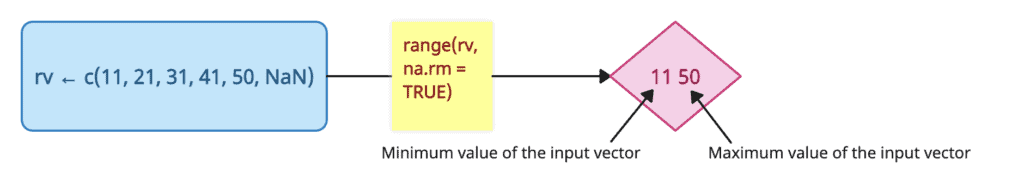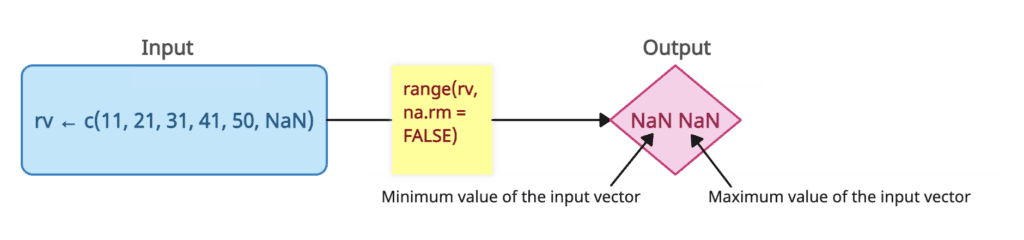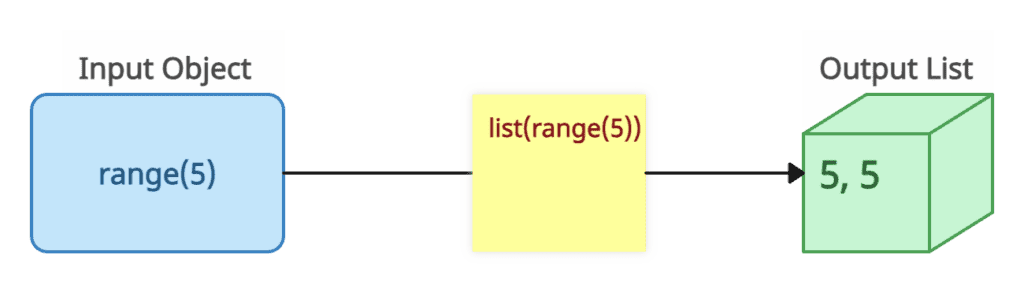# What is the range() Function in R

The range() function in R is used to return a vector with two elements:

1. The first item represents the minimum value of the input vector.
2. The second item represents the maximum value of the input vector.## Syntax

``````range(…, na.rm = FALSE)
``````

## Parameters

1. …: It represents any numeric or character objects or vectors.
2. na.rm: It takes a Boolean value (TRUE or FALSE), suggesting that the NaN (Not a Number) values should be omitted.

## Return value

The range() method returns a vector object holding the result.

## Example 1: How to use the range() function

``````# Creating an input vector object
rv <- c(11, 21, 31, 41, 50, NaN)

# Calling the range() method
range(rv, na.rm = TRUE)
``````

Output

`````` 11 50
``````

## Example 2: Passing na.rm = false``````# Creating an input vector object
a <- c(11, 21, 31, 41, 50, NaN)

# Calling the range() method
range(a, na.rm = FALSE)
``````

Output

`````` NaN NaN
``````

## Example 3: Converting a range object to a listYou can convert a range object to a list using a range() method.

``````data <- range(5)
print(data)
print("After converting a range object to list")
print(list(data))``````

Output

`````` 5 5

 "After converting a range object to list"

[]
 5 5
``````

That’s it!